Courses

# High Pressure Vapour Liquid Equilibria Civil Engineering (CE) Notes | EduRev

## Civil Engineering (CE) : High Pressure Vapour Liquid Equilibria Civil Engineering (CE) Notes | EduRev

The document High Pressure Vapour Liquid Equilibria Civil Engineering (CE) Notes | EduRev is a part of the Civil Engineering (CE) Course Thermodynamics.
All you need of Civil Engineering (CE) at this link: Civil Engineering (CE)

High Pressure Vapour Liquid Equilibria

At relatively high pressures the VLE relations used in the last section lose exactness especially with respect to the activity coefficient-based approach for description of the non-ideal behaviour of the liquid phase. This is because the assumption that the activity coefficients are weakly dependent on pressure no longer remains a realistic approximation. In addition, the gas phase P-V-T behaviour can no longer be described by the truncated virial EOS. Under such conditions a use of a higher order EOS, which may be applied both to the gas and liquid phase is preferred. As we have seen in chapter 2, the cubic EOS provides just that advantage; besides they offer a reasonable balance between accuracy and computational complexity. We start with the general criterion for phase equilibria as applied to vapourliquid systems, given by eqn. 6.127: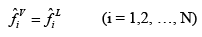..(7.62)

An alternative form of the last equation results from introduction of the fugacity coefficient using eqn. 6.129 and 6.130: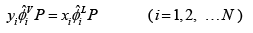..(7.63)

The last equation reduces to: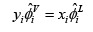..(7.64)

VLE of pure species
For the special case of pure species i, equation 7.64 reduces to: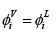....(7.65)
If both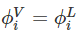are expressed in terms of cubic EOS as defined by any of the eqns. 6.104 to 6.107, for a given T one may obtain the saturation vapour pressure by means of suitable algorithm .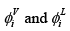VLE from K-value Correlations for Hydrocarbon Systems
Using eqn. 7.64 one can write, Ki = yi /xi
Alternately: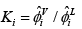...(7.66)

As evident from eqns. 6.155 to 6.157, the expression for species fugacity coefficients for mixtures described by cubic EOS are relatively complex, which in turn makes the estimation of the K-factors difficult as iterative solutions to obtaining T, P and/or compositions are inevitable. As demonstrated in the last section, this is true even for the fugacity and activity coefficient based formulation of the VLE problem. The use of cubic EOS for description of fugacity coefficients of species in both phases poses additional difficulty owing to the intrinsic complexity of the expressions shown in eqns. 6.155 to 6.157.

However, in the case of VLE of light hydrocarbon mixtures a reasonable simplification may be achieved by assuming ideal solution behaviour for both the phases. This is a relatively practical approximation as hydrocarbons being non-polar in nature, the intermolecular interactions are generally weaker than amongst polar molecules. In effect in the case of lighter hydrocarbons (C1-C10) the interactions between the same species and those between dissimilar species are not significantly different. This forms the basis of assuming ideal solution behaviour for such system. It may be noted that since equilibrium pressures in light hydrocarbon systems tend to be ‘high’ (as they are lowboiling) under practical conditions of distillation processes, ideal solution behaviour yields far more accurate results than would be possible by ideal gas assumption.

We develop next the result that obtains owing to the assumption of ideal solution behaviour. The chemical potential of all species in an ideal solution is given by eqn. 6.77: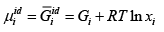...(7.67)

For a real solution: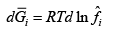...(7.68)

At the same time for pure species at same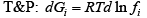...(7.69)

From eqns. (7.68) and (7.69) it follows that: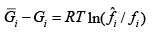...(7.70)
From (7.67)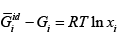...(7.71)

Thus from eqns. (7.70) and (7.71):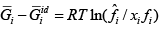...(7.72)

For an ideal solution LHS of (7.72) is identically zero; hence for such a solution: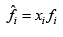...(7.73)

For a real gas mixture the fugacity coefficientφˆi is defined by: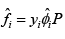In analogy, for a real solution we defineφ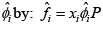Or: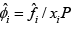...(7.74)

Using (7.74) for an ideal solution: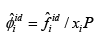...(7.75)

Using (7.73) in (7.75) it follows: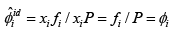...(7.76)

Thus for an ideal solution: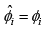...(7.76)

Now considering the light hydrocarbon systems, the application of eqn. 7.76 in 7.66 gives: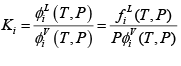...(7.77)

Using eqn. 6.119 we substitute for the fugacity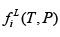Thus: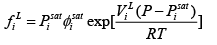Where,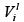is the molar volume of pure species i as a saturated liquid. Thus the K-value is given by: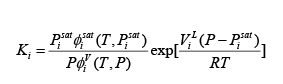...(7.78)

The advantage of eqn. 7.78 is that it is a function of the properties of the pure species only, and therefore its dependence on composition of the vapour and liquid phases is eliminated. The K-factor then is a function of temperature and pressure alone. The terms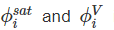in eqn. 7.78 can in principle be computed using expression provided by cubic EOS (i.e., eqns. 6.104 – 6.107) or a corresponding expression from an higher order EOS, including the generalized correlation (section 6.9). This allows K-factors for light hydrocarbons to be as functions of T and P.

However, it may be noted that the computation of fugacities at high pressures (and/or temperatures) can potentially be rendered difficult as above the critical temperature the liquid state is necessarily hypothetical, while at pressures higher than the saturation pressure the vapour state is hypothetical. This is corrected for by some form of extrapolations to those hypothetical states. Various approaches have been described in the literature  The nomographs of K-factors (see figs. 7.11 and 7.12) reported by Dadyburjor provide an example of one such approach.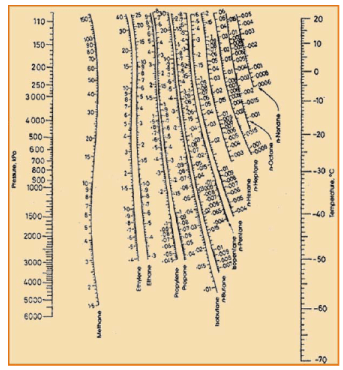Fig. 7.11 K-factors in light hydrocarbon systems (low temperature range)
[Source: Dadyburjor; D.B., Chem. Eng.Progr., Vol. 74 (4) pp.85-86 (1978)].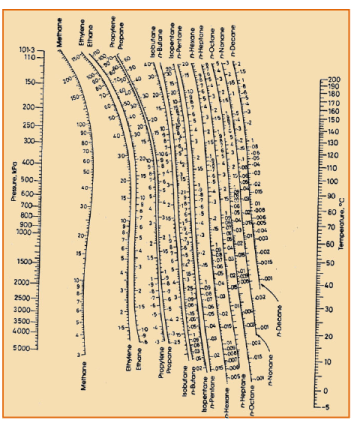Fig. 7.12 K-factors in light hydrocarbon systems (high temperature range)

The nomographs may be conveniently used purpose of VLE calculations in hydrocarbon systems as they the K-factors for each species can be estimated at a given T and P. This is done by drawing a straight line connecting the given temperature and pressure; the corresponding Ki value is read off from the point of intersection of this line with the Ki curve for a particular species. For bubble point (either T or P) calculations one uses: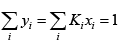.....(7.79)

•  For pressure calculation: If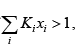assumed pressure is lower than the correct value;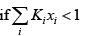the assumed pressure is higher than the correct pressure. Thus, pressure needs to be revised for the next step of calculation.
• Similarly, for temperature calculation: if, assumed temperature is higher than the  correct value;the assumed temperature is lower than the correct value. Thus, temperature needs to be revised for the next step of calculation.

On the other hand the solution for dew point calculations derives from:.....(7.80)

• For pressure calculation:assumed pressure is higher than the correct value; ifthe assumed pressure is lower than the correct pressure. Thus, pressure needs to be revised for the next step of calculation.
• Similarly, for temperature calculation: ifassumed temperature is lower than its  correct value; ifthe assumed temperature is higher than its correct value. Thus, temperature needs to be revised for the next step of calculation.

The use of these equations illustrated below using an example.

High Pressure VLE using cubic EOS

This constitutes a generalized approach without any simplifying assumptions such as employed for light hydrocarbons. The governing relation thus is eqn. 7.66.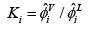The fugacity of each species, either in vapour or liquid phase, is computed using the expressions that apply to use of cubic EOS (eqns. 6.155 to 6.157). For relevant VLE calculations once again the eqns. 7.79 and 7.80 are employed. The steps for computing (for example) the bubble pressure are enlisted below. The basic principle used for other types of standard calculations (such as discussed for low to moderate pressure VLE systems, table 7.1) remains the same.

Bubble pressure algorithm:

Given T and {x} , to calculate P and { y }

a) Solve for P and { y } first by assuming Raoult’s Law algorithm for bubble pressure

b) Using solution in ‘a’ estimate {K } using eqn. 7.66 with the given values of T and {x } ; and the latest values of P and { y }

c) Next calculate {Kx } and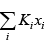d) Calculate all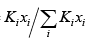e) Using normalized { y} , recalculate {Ki } and ii i ∑ Kx
f) Haschanged between steps ‘c’ and ‘e’? If yes return to step ‘d’

g) Ifhas not changed between two successive iterations between steps ‘c’ and ‘e’ is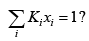h) If yes, the last values of P and { yi ≡ Kixi} give the final bubble temperature Pb (f) ,and vapour compositions.

i) If no, and last iithen Plast < Pb(f) ; revise to new P as: new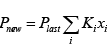and return to  step (c).and return to step ‘b’.

j) If no, and last iithen Plast < Pb(f)  revise to new P as: newand  return to step (c).and return to step ‘b’.

Offer running on EduRev: Apply code STAYHOME200 to get INR 200 off on our premium plan EduRev Infinity!

## Thermodynamics

56 videos|92 docs|33 tests

,

,

,

,

,

,

,

,

,

,

,

,

,

,

,

,

,

,

,

,

,

;